# Surface Area Calculator

## Surface Area for Cylinder Calculator, Rectanglar Prism Calculator, Triangular Prism Calculator, Cone Calculator, Cube Calculator, Cuboid Calculator, Triangular Pyramid Calculator, Rectangular Pyramid Calculator, Sphere Calculator with Surface Area Formula

HomeMath

Surface Area Calculator

=

## Surface Area of Cylinder Formula

### Surface Area of Solid Cylinder Formula

#### r is radius, h is height and Pi value is 3.14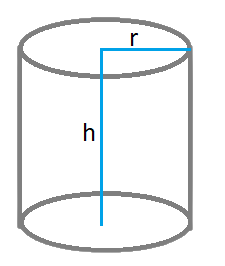### Surface Area of a Hollow Cylinder

#### R is outer radius, r is inner radius, h is height and Pi value is 3.14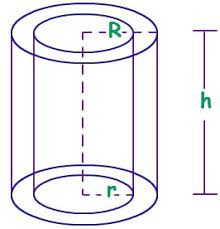## Surface Area of Sphere formula

### Formula to calculate Surface Area of a Sphere using Radius

#### r is radius and Pi value is 3.14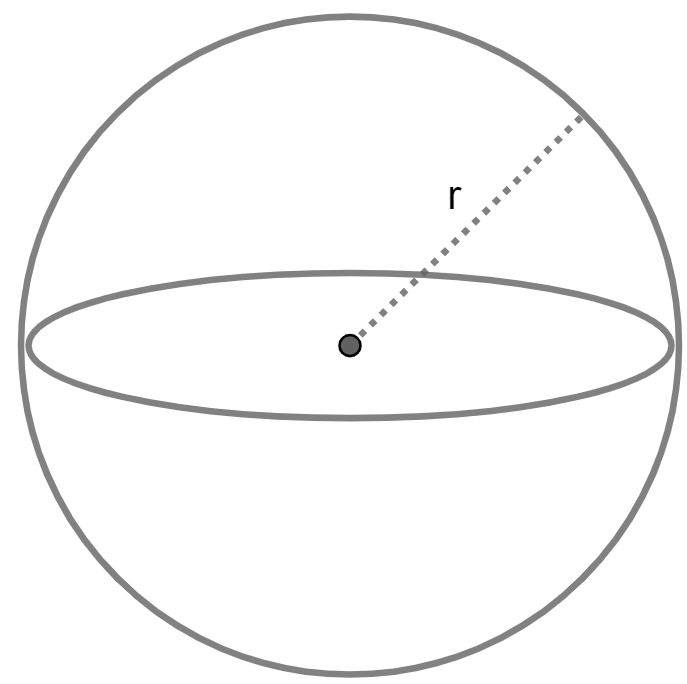## Surface Area of Hemisphere Formula

### Formula to calculate Surface Area of a Hemisphere using Radius

#### r is radius and Pi value is 3.14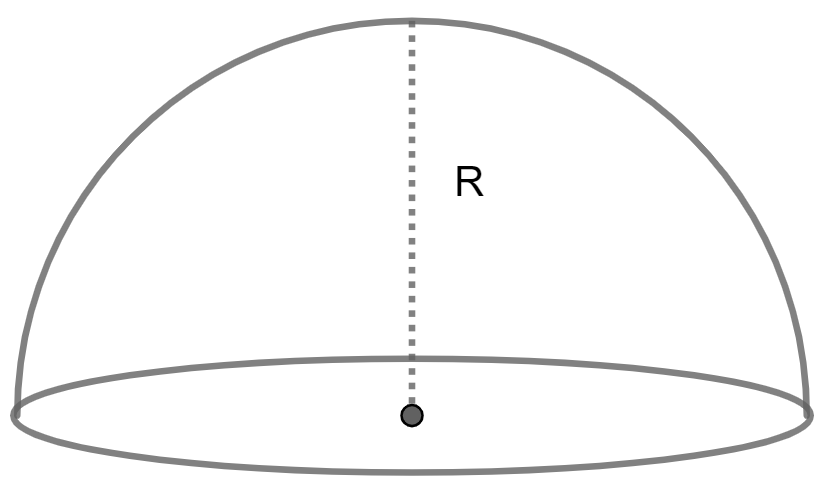## Surface Area of Cone Formula

### Formula to calculate Surface Area of a Cone using Radius and Height

#### r is radius, h is height and Pi value is 3.14## Surface Area of Cube Formula

### Formula to calculate Surface Area of a Cube using Edge

#### a is edge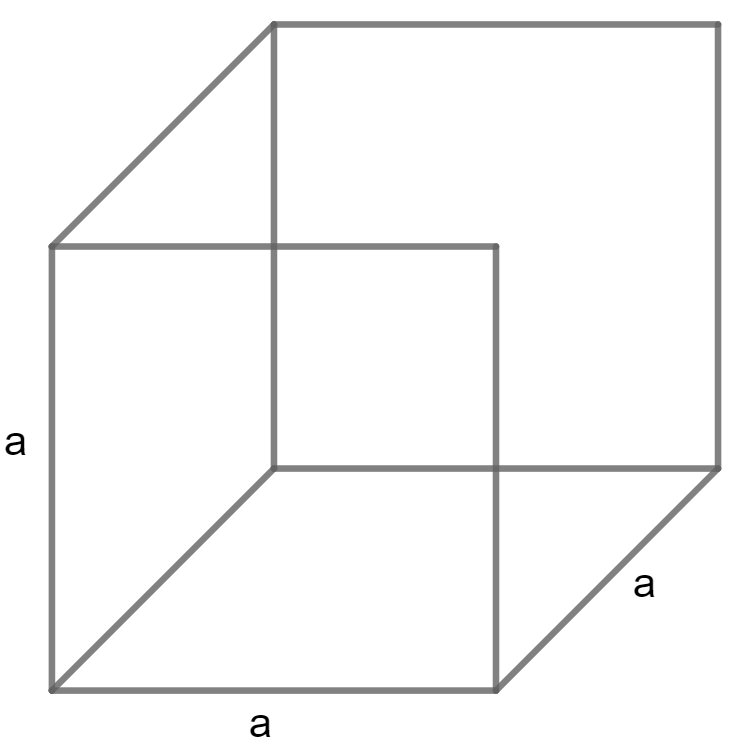## Surface Area of Cuboid Formula

### Formula to calculate Surface Area of a Cuboid using Length, Width and Height

#### L is Length, W is Width, and H is Height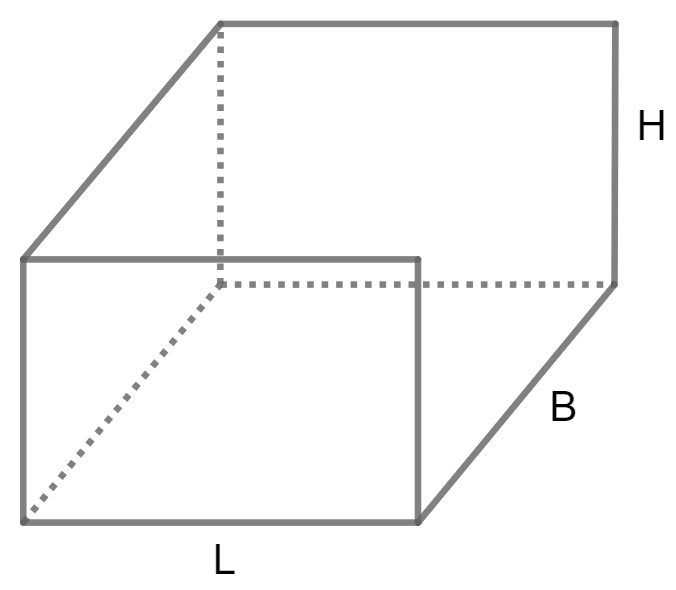## Pyramid Volume Formula

### Formula to calculate Surface Area of a Triangular Pyramid using Base, Slant Height and Height

#### A is Base Height, S is Slant Height and B is Base edge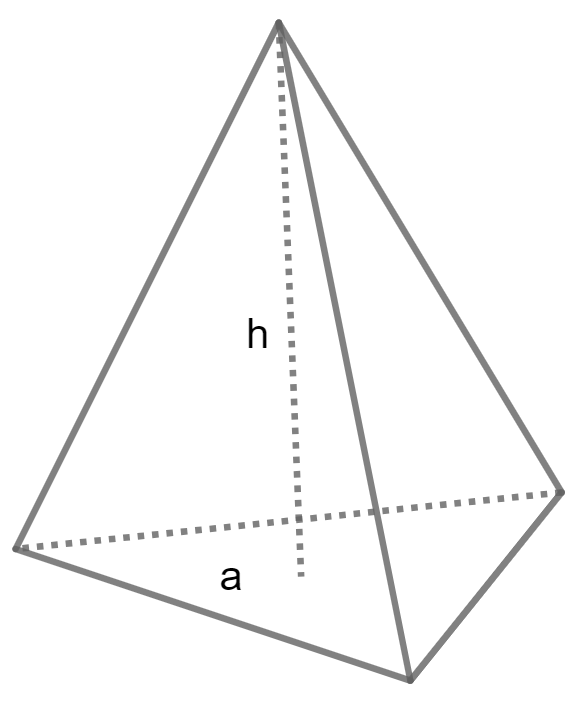### Formula to calculate Surface Area of a Square Pyramid using Base edge and Height

#### A is base edge and H is Height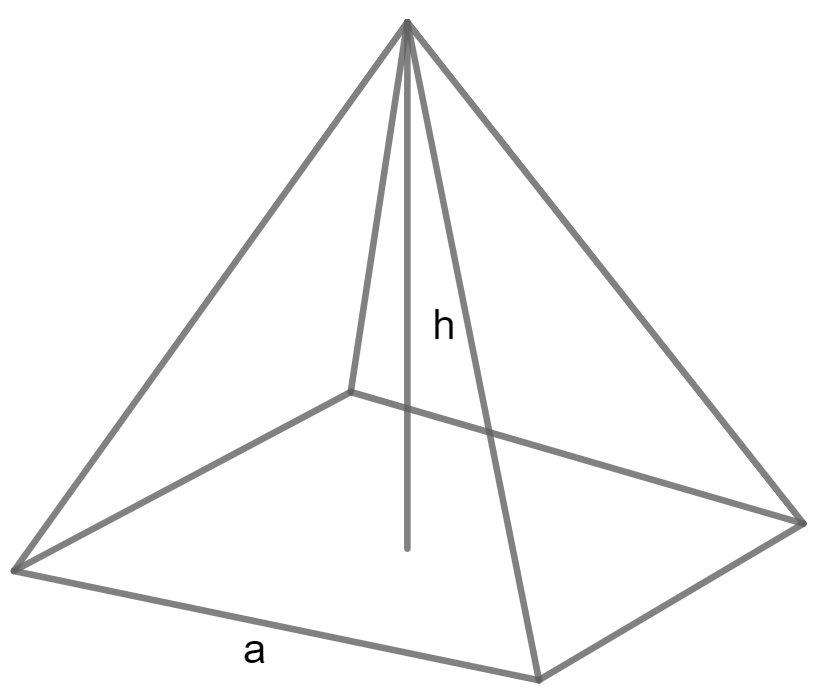### Formula to calculate Surface Area of a Pentagonal Pyramid using Base, Slant height and Height

#### A is Base Height, S is Slant Height and B is Base edge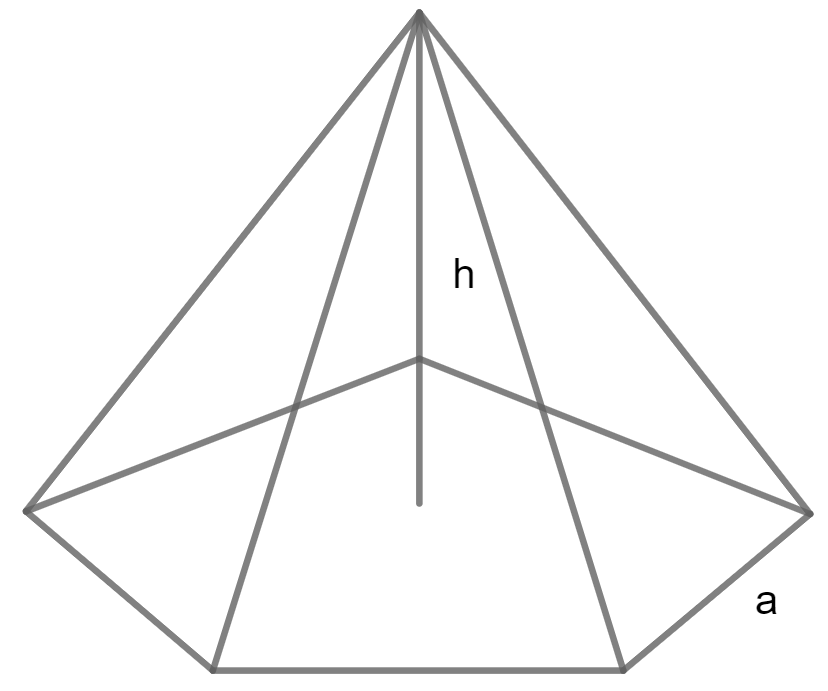### Formula to calculate Surface Area of a Rectangular Pyramid using Base, Width and Height

#### L is base length, W is Width and H is Height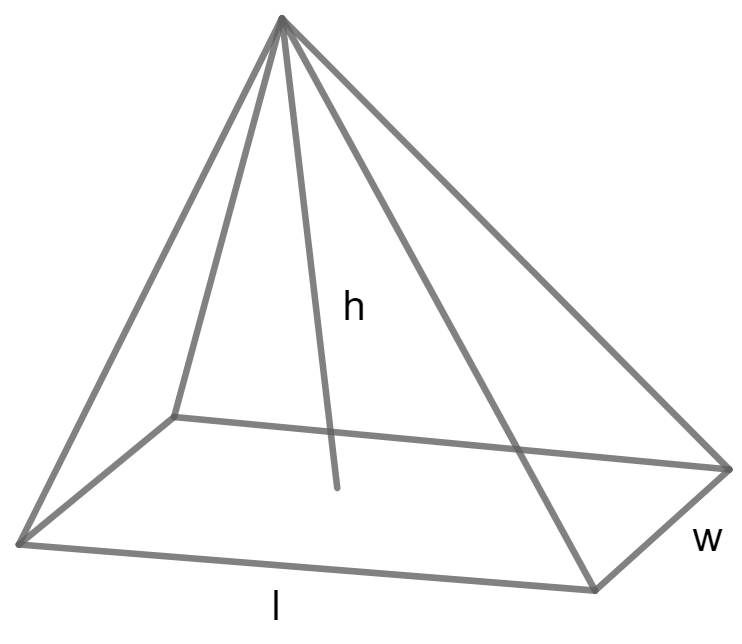## Surface Area of Prism Formula

### Formula to calculate Surface Area of a Triangular Prism using Base, Length and Height

#### B is Base and H is Height and L is Length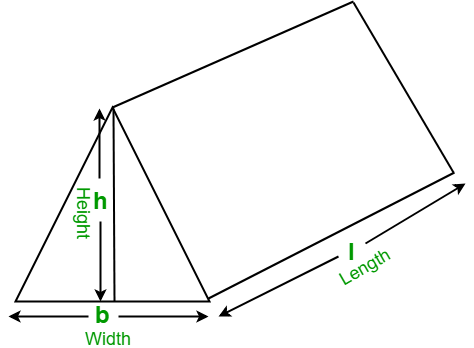### Formula to calculate Surface Area of a Square Prism using Base edge and Height

#### A is base edge and H is Height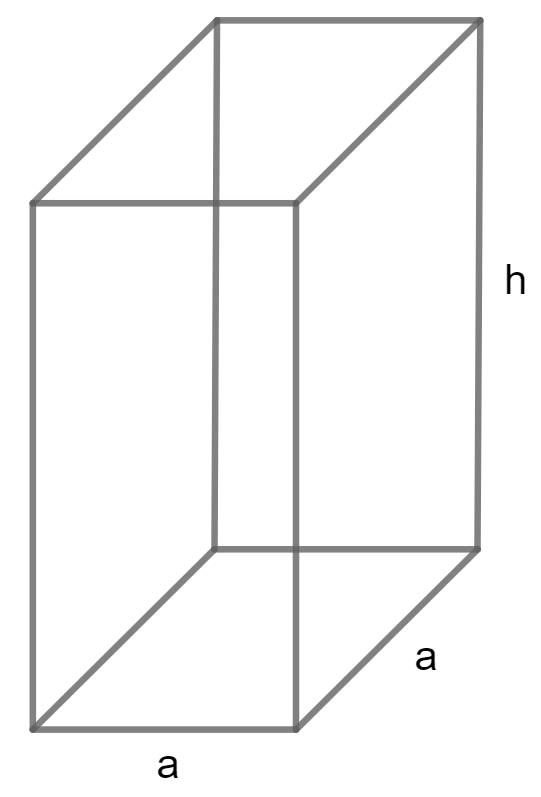### Formula to calculate Surface Area of a Rectangular Prism using Width, Length and Height

#### W is Width and L is Length and H is Height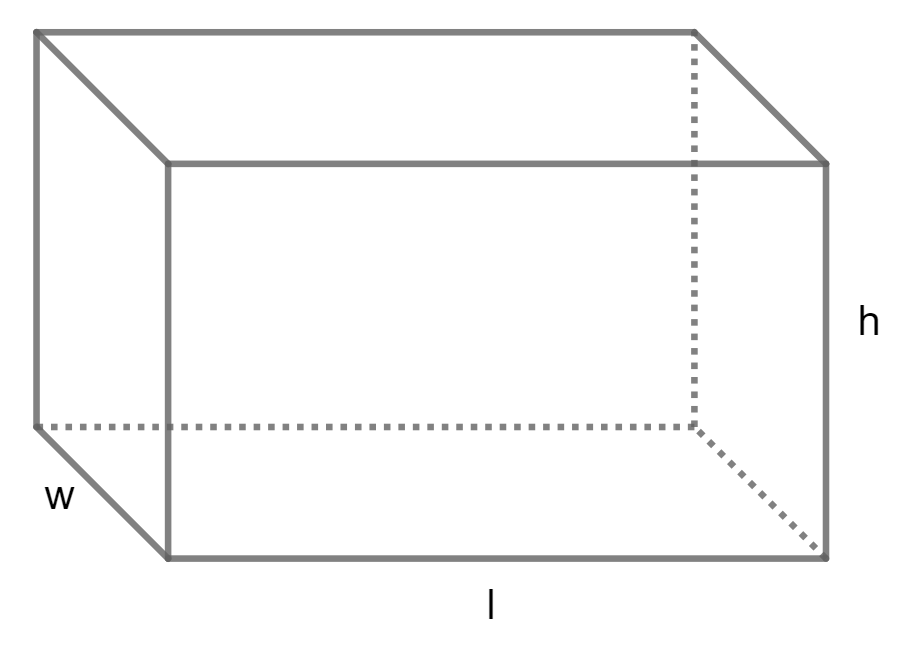### Formula to calculate Surface Area of a Pentagonal Prism using Base edge and Height

#### B is base edge, A is Apothem and H is Height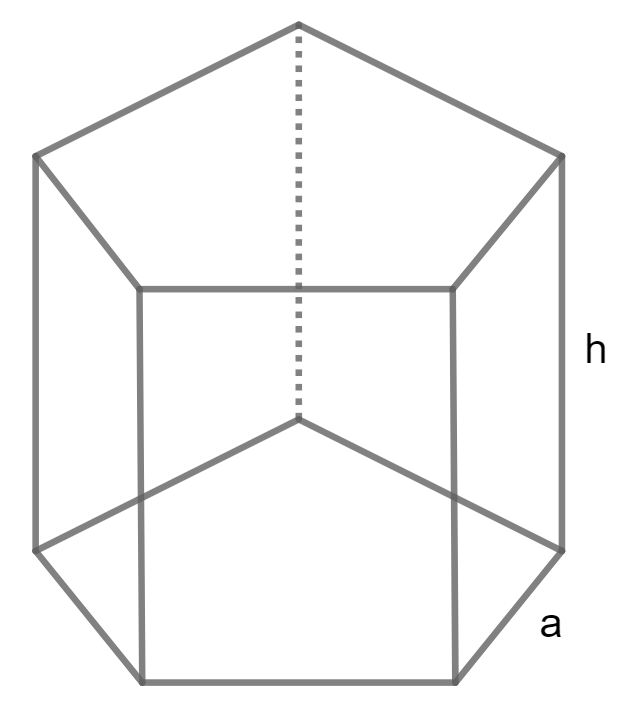### Formula to calculate Surface Area of a Hexagonal Prism using Base edge and Height

#### A is base edge and H is Height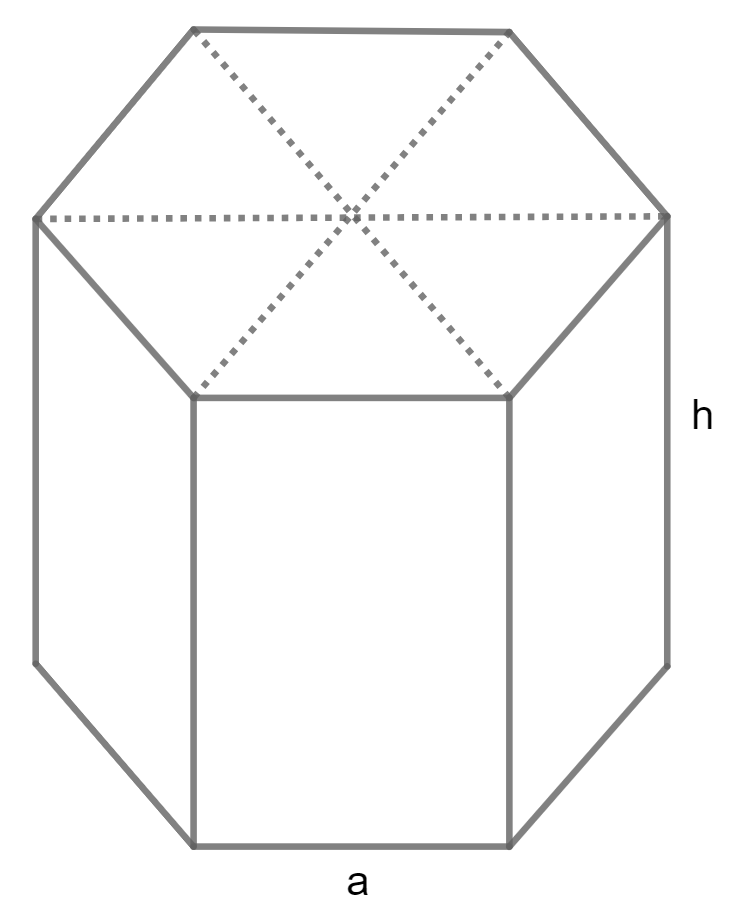## Ellipsoid Surface Area Formula

### Formula to calculate Surface Area of a Ellipsoid using Axes

#### A, B and C are axes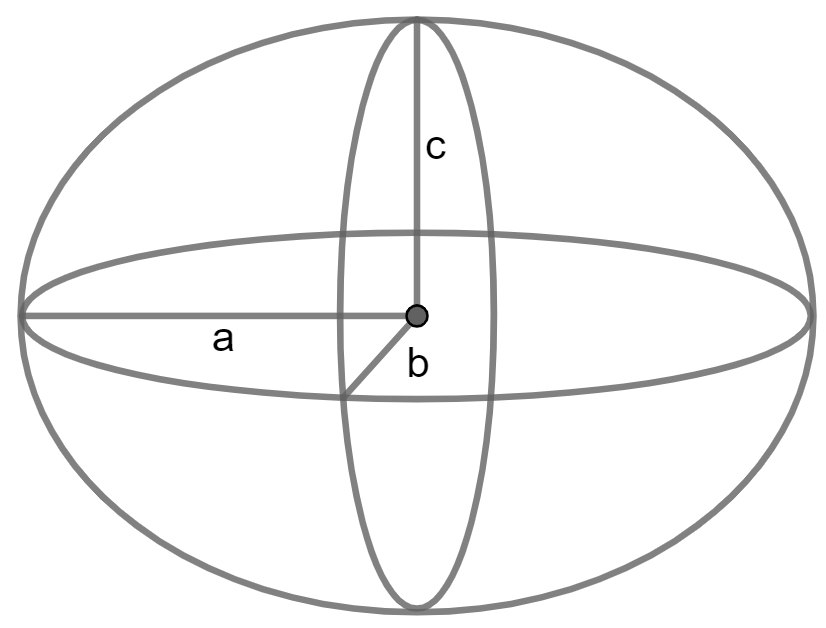## Tetrahedron Surface Area Formula

### Formula to calculate Surface Area of a Tetrahedron using Edge

#### a is Edge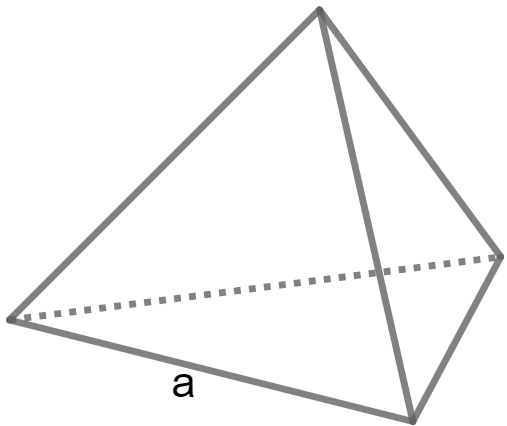## Hollow Cylinder Surface Area Formula

### Surface Area of a Hollow Cylinder

#### R is outer radius, r is inner radius, h is height and Pi value is 3.14## Spherical Cap Surface Area Formula

### Formula to calculate Surface Area of a Spherical Cap using Radius and Height

#### H is Height, R is Radius and Pi value is 3.14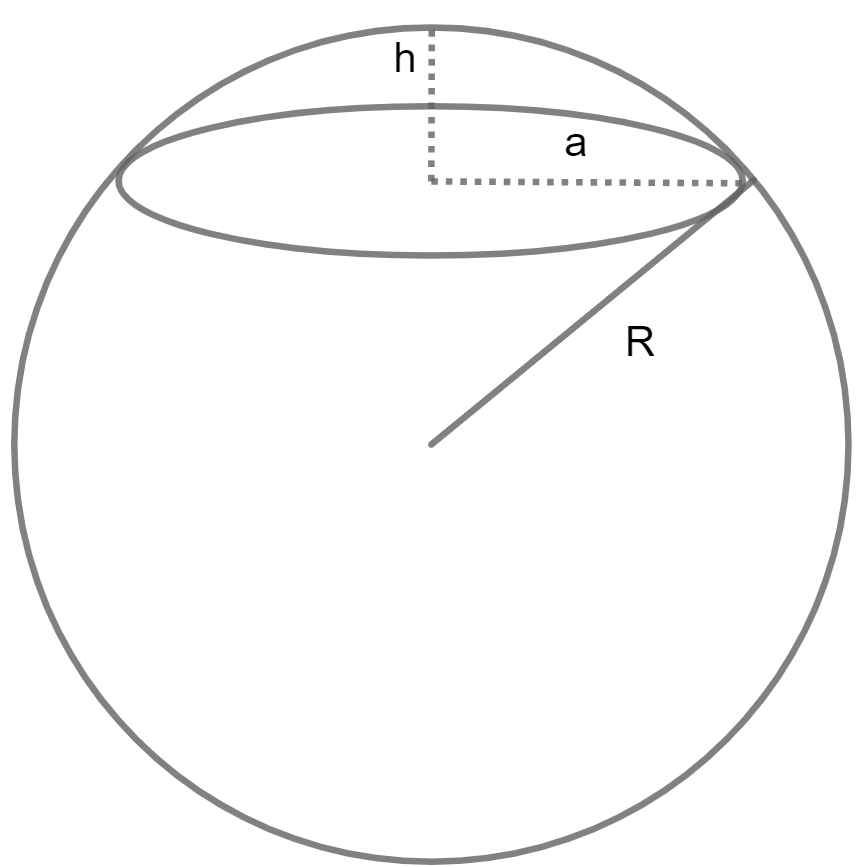## Frequently Asked Questions on Surface Area Calculator and Surface Area Formula